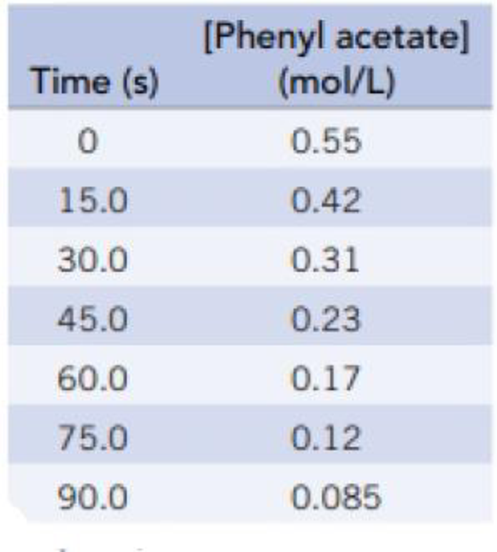Chapter 14, Problem 6PS

Chapter
Section
Textbook Problem

Phenyl acetate, an ester, reacts with water according to the equationThe data in the table were collected for this reaction at 5 °C.(a) Plot the phenyl acetate concentration versus time, and describe the shape of the curve observed. (b) Calculate the rate of change of the phenyl acetate concentration during the period 15.0 seconds to 30.0 seconds and also during the period 75.0 seconds to 90.0 seconds. Why is one value smaller than the other?

(a)

Interpretation Introduction

Interpretation:

A plot has to be drawn for the concentration versus time of phenyl acetate and the shape of the curve has to be described.

Concept Introduction:

Relative rates and stoichiometry: During a chemical reaction, amounts of reactant decrease with time and amounts of products increases.

Reaction Rate = - 1coefficientΔ[reactants]Δt = +1coefficient Δ[products]Δt

Explanation

The plot of concentration versus time of phenyl acetate is given as,

Figure 1

(b)

Interpretation Introduction

Interpretation:

The rate of change of Phenyl Acetate concentration during the given time intervals has to be calculated and the reason why one value is smaller than other has to be explained.

Concept Introduction:

Relative rates and stoichiometry: During a chemical reaction, amounts of reactant decrease with time and amounts of products increases.

Reaction Rate = - 1coefficientΔ[reactants]Δt = +1coefficient Δ[products]Δt

Still sussing out bartleby?

Check out a sample textbook solution.

See a sample solution

The Solution to Your Study Problems

Bartleby provides explanations to thousands of textbook problems written by our experts, many with advanced degrees!

Get Started

Find more solutions based on key concepts